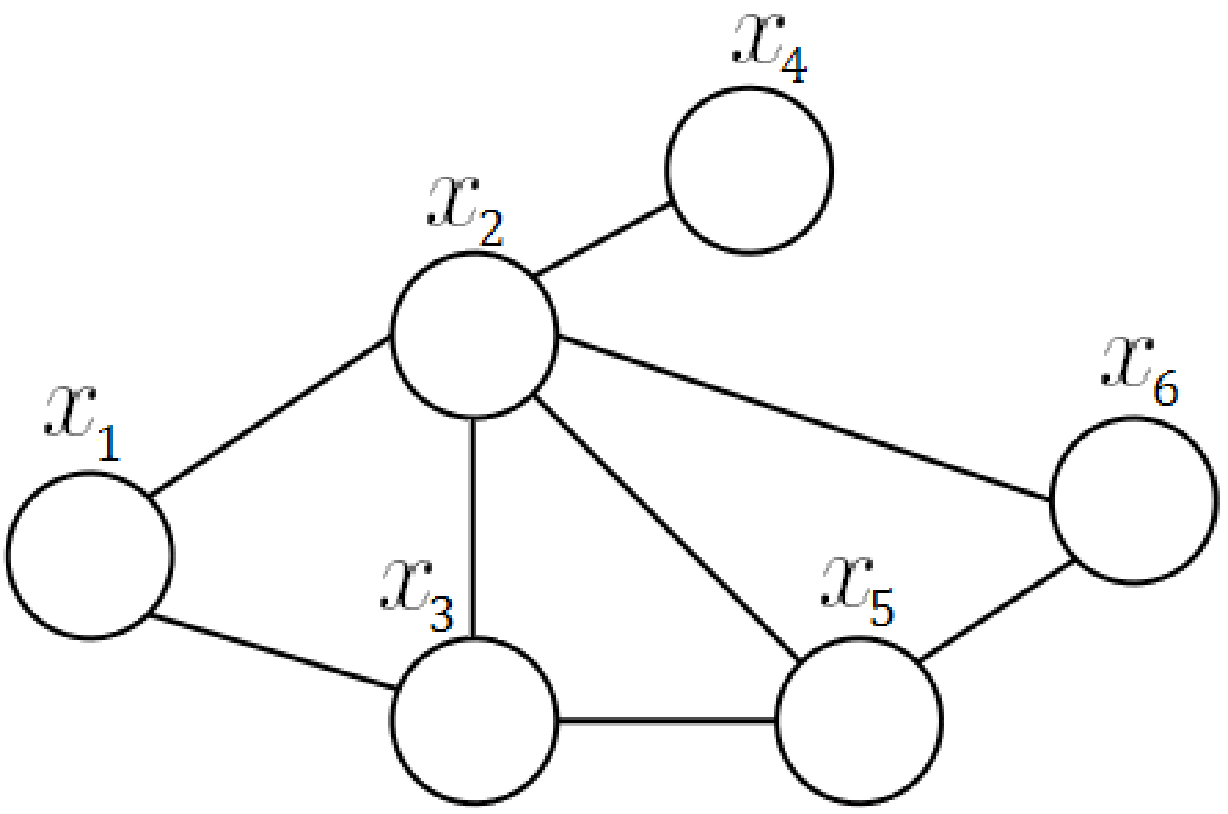# Junction Tree local consistency and global consistency

This note is to describe the Junction Tree local consistency and global consistency (text book:  P109, Example 6.1)

1. Reference:
1. pgm_Princeton_COS513 Foundations of Probabilistic Modelinglecture7.pdf
2. gouws_python_2010: a master thesis on how to implement graphical model with python
3. Text: Bayesian Reasoning and Machine Learning

1. Junction tree property(JTP): For each pair U, V of cliques with intersection S, all cliques on the path between U and V contain S. (from gouws_python_2010.pdf, a master thesis on how to implement graphical model with python)
2. Example 1 to reflect the propertyAdd Separators in diagram b), you may find it comply with JTP

->1. Example 2:  another good example to illustrate Junction tree property:

1. Original diagram1. The above diagram can be represented by below two Cliques graph1. According to JTP, the diagram (b) does not comply with JTP.  As node {x5} is the intersection of C2 and C4.  However, the path between C2 and C4 contains C3, which do not contain x5.
2. For diagram (a), node {x5} stays in C2 and C4, which no more cliques (except c2 and c4) between the path C2 and C4. Similar for node {x2} in diagram (a), node {x2} is the intersection of C3 and C4. The path between C3 to C4 also contains cliques C2, which has node {x2}. You can double check all others intersect, they comply with the JTP rule.

« (Previous News)